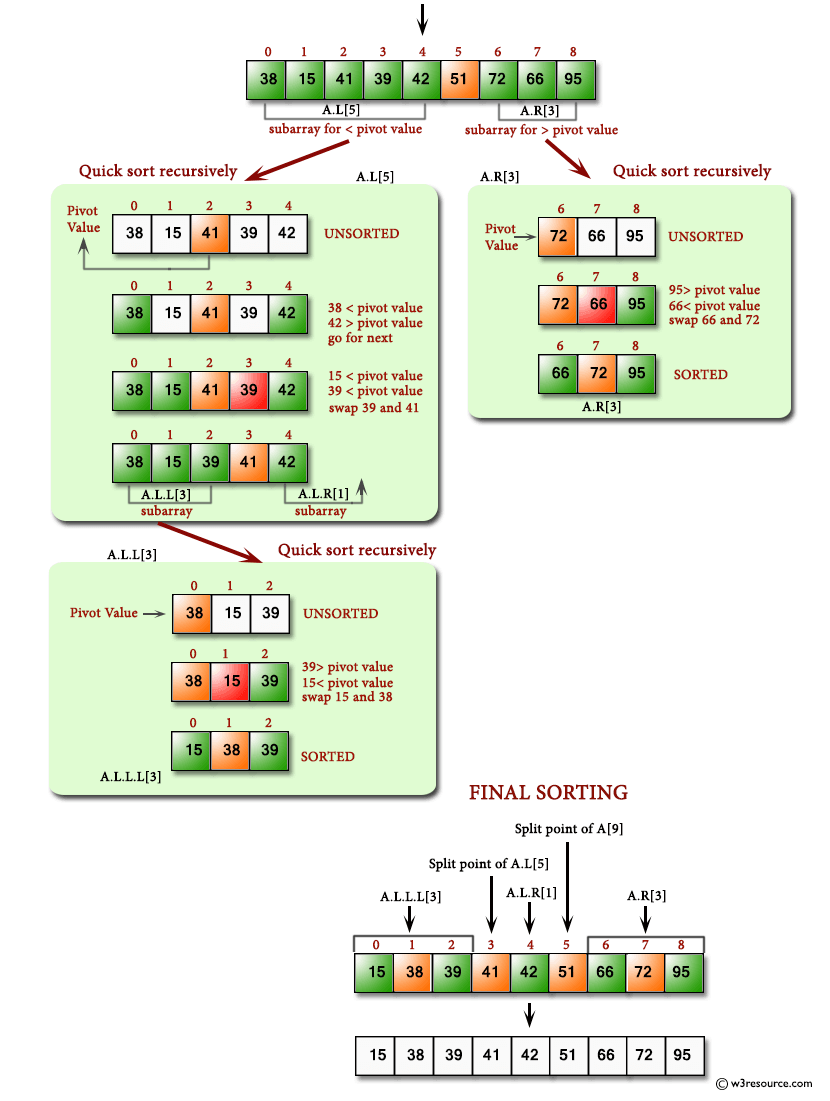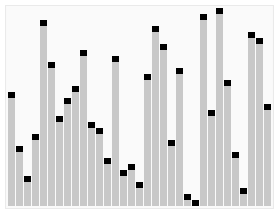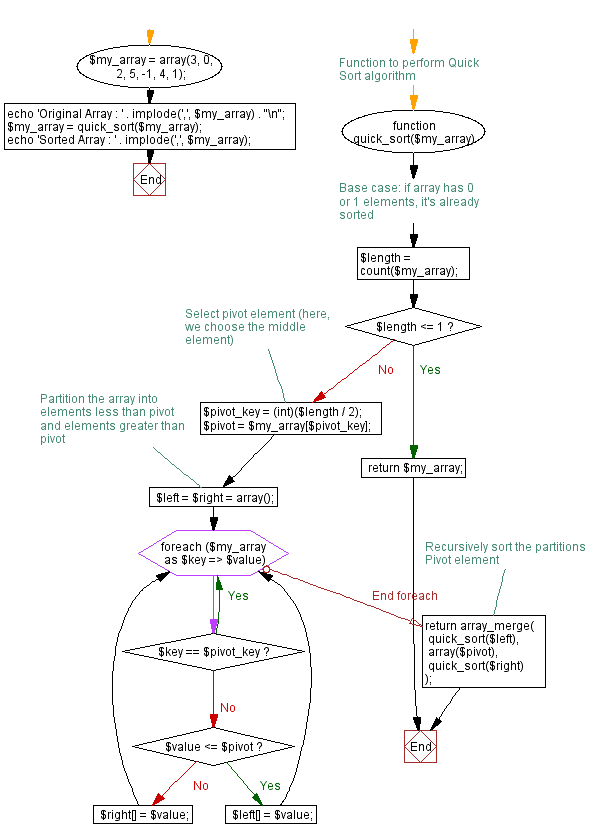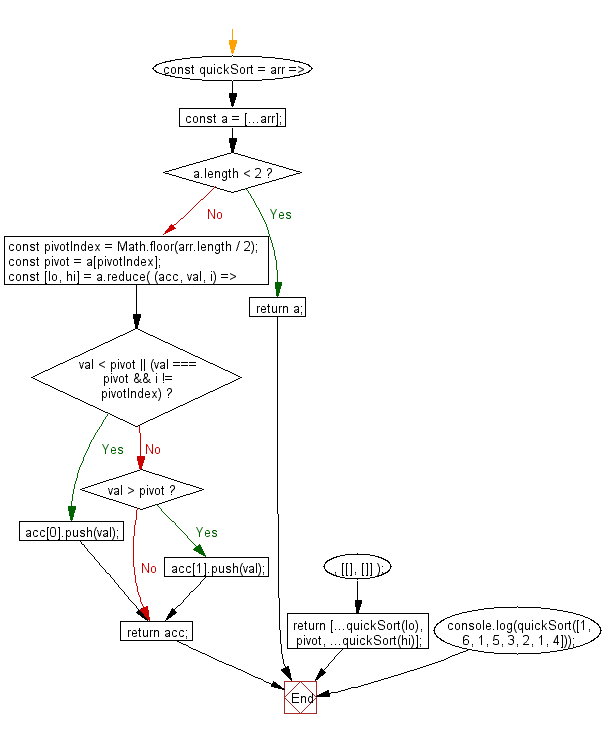# JavaScript Searching and Sorting Algorithm: Sorts an array of numbers, using the quicksort algorithm

## JavaScript Searching and Sorting Algorithm: Exercise-1 with Solution

Write a JavaScript program to sort a list of elements using Quick sort.

Quick sort is a comparison sort, meaning that it can sort items of any type for which a "less-than" relation (formally, a total order) is defined.

Pictorial presentation - Quick Sort algorithm :Animated visualization of the quicksort algorithm. The horizontal lines are pivot values. Animation credits: RolandH

Sample Solution-1:

JavaScript Code:

``````function quick_Sort(origArray) {
if (origArray.length <= 1) {
return origArray;
} else {

var left = [];
var right = [];
var newArray = [];
var pivot = origArray.pop();
var length = origArray.length;

for (var i = 0; i < length; i++) {
if (origArray[i] <= pivot) {
left.push(origArray[i]);
} else {
right.push(origArray[i]);
}
}

return newArray.concat(quick_Sort(left), pivot, quick_Sort(right));
}
}

var myArray = [3, 0, 2, 5, -1, 4, 1 ];

console.log("Original array: " + myArray);
var sortedArray = quick_Sort(myArray);
console.log("Sorted array: " + sortedArray);
```
```

Sample Output:

```Original array: 3,0,2,5,-1,4,1
Sorted array: -1,0,1,2,3,4,5
```

Flowchart:Sample Solution-2:

• Use recursion.
• Use the spread operator (...) to clone the original array, arr.
• If the length of the array is less than 2, return the cloned array.
• Use Math.floor() to calculate the index of the pivot element.
• Use Array.prototype.reduce() and Array.prototype.push() to split the array into two subarrays (elements smaller or equal to the pivot and elements greater than it), destructuring the result into two arrays.
• Recursively call quickSort() on the created subarrays.

JavaScript Code:

``````const quickSort = arr => {
const a = [...arr];
if (a.length < 2) return a;
const pivotIndex = Math.floor(arr.length / 2);
const pivot = a[pivotIndex];
const [lo, hi] = a.reduce(
(acc, val, i) => {
if (val < pivot || (val === pivot && i != pivotIndex)) {
acc.push(val);
} else if (val > pivot) {
acc.push(val);
}
return acc;
},
[[], []]
);
return [...quickSort(lo), pivot, ...quickSort(hi)];
};

console.log(quickSort([1, 6, 1, 5, 3, 2, 1, 4]));
```
```

Sample Output:

```[1,1,1,2,3,4,5,6]
```

Flowchart:Live Demo:

See the Pen searching-and-sorting-algorithm-exercise-1 by w3resource (@w3resource) on CodePen.

* To run the code mouse over on Result panel and click on 'RERUN' button.*

Improve this sample solution and post your code through Disqus

What is the difficulty level of this exercise?

﻿

## JavaScript: Tips of the Day

Errors

```const name = 'Owen';

console.log(name());
```

The variable name holds the value of a string, which is not a function, thus cannot invoke.
TypeErrors get thrown when a value is not of the expected type. JavaScript expected name to be a function since we're trying to invoke it. It was a string however, so a TypeError gets thrown: name is not a function!
SyntaxErrors get thrown when you've written something that isn't valid JavaScript, for example when you've written the word return as retrun. ReferenceErrors get thrown when JavaScript isn't able to find a reference to a value that you're trying to access.

Ref: https://bit.ly/3jFRBje